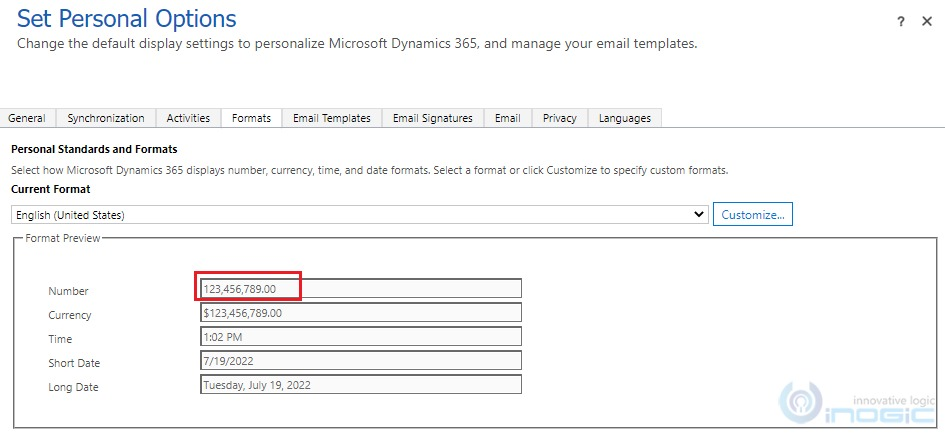# Format input values into different user input formats using Formatting API in PCF Control

Introduction

In the previous blog, we have explained the Formatting API of PCF Control where we used the Formatting API to format the input values.

Earlier, we used different Formatting API methods to format the number and date values. In this blog, we will introduce a new Formatting API method (formatUserInput) that formats the given input values in different user input formats.

In the below example, we have set the user settings as Number with 2 precision:Given below are the two (Decimal Number) examples, where in the first example, the input value format to the user format is defined in user settings of Dynamics 365 CRM. And in the second example, the input value format to the user input format is passed as a input parameter to Formatting API method (formatUserInput).

`//Function that return result in user decimal format from input...`